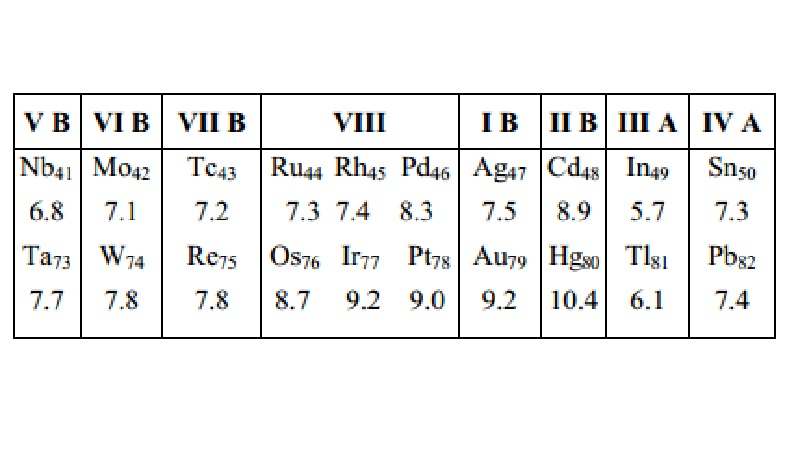Home | | Chemistry | | Chemistry | Anomalous periodic properties in terms of screening constant, stability etc.

# Anomalous periodic properties in terms of screening constant, stability etc.According to Hund's rule atoms having half-filled or completely filled orbitals are comparatively more stable and hence more energy is needed to remove an electron from such atoms. The ionization potentials of such atoms are, therefore, relatively higher than expected normally from their position in the periodic table.

Anomalous periodic properties in terms of screening constant, stability etc.

According to Hund's rule atoms having half-filled or completely filled orbitals are comparatively more stable and hence more energy is needed to remove an electron from such atoms. The ionization potentials of such atoms are, therefore, relatively higher than expected normally from their position in the periodic table.

Example

A few irregularities that are seen in the increasing values of ionization potential along a period can be explained on the basis of the concept of half-filled and completely filled orbitals, e.g., Be and N in the second period and Mg and P in the third period have slightly higher values of ionization potentials than those normally expected. This is explained on the basis of extra stability of the completely-filled 2s-orbital in Be(Be2s2) and 3s-orbital in Mg (Mg3s2) and of half-filled 2p-orbital in N (N2s2p6) and 3p-orbital in P (P3s2p3).

Another example for irregularity in Ionization potential is observed in the case of B and Be.

Ionization energy of boron (B 2s22p1) is lower than that of beryllium (Be 2s2) [B = 8.3 eV, Be = 9.3 eV], since in case of boron we have to remove a 2p1 electron to get B+[B (2s2p1) B+(2S2) + e-] while in case of Be we have to remove a 2s1 electron of the same main energy level to have Be+ ion. [Be (2s2) Be+(2s1) +e-].

There is an exception to the vertical trend of ionization potential. This exception occurs in the case of those elements whose atomic numbers are greater than 72. Thus the ionization potentials of the elements from Ta73 to Pb 82 are greater than those of the elements of the same sub-group above them as shown below : (First ionization potential values are given in electron volts, eV).

The reason for the abnormal behaviour (i.e. an increase in the value of I1 from Nb Ta, Mo W, �..,Sn Pb) shown by the elements from Ta73 to Pb82 is due to the lanthanide contraction as a result of which there occurs an increase in the nuclear charge without a corresponding increase in size through the rare earths. In fact, the size actually decreases in this region.

Periodic Variations

Similarly in moving down a group electron affinity values generally decrease, e.g. ECl>EBr>EI. This is due to the steady increase in the atomic radius of the elements.

Exceptions

There are, however, some exceptions to this general rule as is evident from the following examples:

It is known that EF < ECl (EF = 322 kJ mol-1 , ECl = 349 kJ mol-1). The lower value of E for F is probably due to the electron-electron repulsion in relatively compact 2p-orbital of F-atom.

In period, electron affinity values generally increase on moving from left to right in a period in the periodic table.

Exceptions

There are, however, exceptions also to this general rule; e.g.

Be and Mg have their EA values equal to zero. Since Be and Mg have completely filled s-orbitals (Be 2s2, Mg 3s2), the additional electron will be entering the 2p-orbital in case of Be and 3p-orbital in case of Mg which are of considerably higher energy than the 2s-and 3s orbitals respectively.

Study Material, Lecturing Notes, Assignment, Reference, Wiki description explanation, brief detail

Related Topics

Copyright © 2018-2021 BrainKart.com; All Rights Reserved. (BS) Developed by Therithal info, Chennai.